# Size Factors

When playing Supremacy 1914 you want to be smart about army composition and how to group them into armies. One major reason for this is the size factor feature.

When an army reaches a certain size, each additional unit of the same type added to the stack only adds a fraction of its damage to the total damage of the army. Size factors are written in this fashion:

a0=1, a25=0.5, a50=0

The above example means that starting at 0 units each additional unit adds 100% of its damage. But starting at 25 units each additional unit only adds 50% of its damage. Finally, starting at 50 units each additional unit adds 0% of its damage to the total damage of the army. This is why splitting up armies often is a good strategy for maximizing damage output.

Here's an overview about all units and their respective size factors: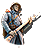Infantry a0=1,a5=0.3,a15=0.1,a40=0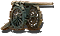Artillery a0=1,a50=0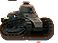Tank a0=1,a5=0.6,a10=0.3,a25=0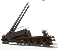Railgun a0=1,a50=0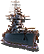Battleship a0=1,a50=0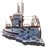Submarine a0=1,a50=0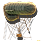Balloon a0=1,a50=0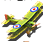Fighter a0=1,a50=0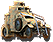Armoured Car a0=1,a6=0.6,a15=0.2,a25=0.1,a40=0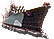Light Cruiser a0=1,a50=0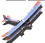Bomber a0=1,a50=0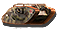Heavy Tank a0=1,a5=0.5,a10=0.2,a25=0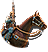Cavalry a0=1,a6=0.6,a15=0.2,a25=0.1,a40=0

••if you attack with 2 x 20 stacks and the defender has 30, does each 20 stack face a defensive attack or just one defensive response per hour/15 minutes?

•So you can only have 4 infantry in a stack before getting a severe penalty? That's duuumb. It should be set like the example for infantry....up to 25 infantry before you start incurring a penalty, and at that point it should drop to only .5 damage. The example rates are what it should be for the infantry. What is currently listed for infantry is far too restrictive and annoying.

•My question about this has always been, say a stack of 40 vs 60 vs 80 troops. Which is better. I think the 40 would be inferior because it would drop below that optimum level after the first lost. 60 would keep you at that level longer. 80 even longer but with 80 would the extra troops sustain damage that is not necessary. Does a stack just need to be big enough to stay above 40 until you can reinforce it ?

•One thing is the damage and another is the force that means "resistance" in this context.

Of course, 80 troops is better than 60 troops, and those are better than 40 troops. However, as the article suggests, 40 troops plus some cavalry, tanks and armored cars would make more damage and could beat a 80 troops' army because more soldiers doesn't mean more damage.

That's important to know because, for example, big armies groups can be obliterated as far as the enemy can keep the melée and send constant stacks of units with different features.

•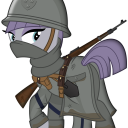The article talks about maximising damage only. The part about the group taking casualties is another story. But yes, it is preferable to have more units so the damage is used in the most efficient way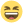So maybe, get a 50 stack artillery and let them shoot, then get a separate 50 stack artilery to do damage again! If you are worried the enemy will focus on these artillery, simply just add a few artillery in the group so they still do maximum damage.

•I think the point is to 'MIX' your stack with several other unit types...this is going to do max damage, remembering that each unit = 1000 < so the battle front can only be so wide when attacking a fort, and this is reason for cap on damage any stack can do,.

Hit Points is part of the dispersion of damage which is done, 60 inf units when cap of damage is 40, perhaps 10 units die and are replace in next tick of melee and your stack is down to 50 inf but still doing max cap of damage of 40

Keeping a Mixed stack of inf, cav, arty,balloons, tanks you are dealing more damage and your damage taken is dispersed.

•Que sucede con la artilleria ? A partir de 50 unidades de artilleria el daño no es acumulativo?

•is that 25 units of each or 25 units total?

•each number is how many units can be in one stack on whatever type of unit before they don't do any more damage

•This makes no sense

•this pretty much just tells you how many units of one type can in a single stack before they don't add anymore damage

•